## Midpoint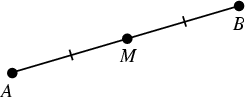The point on a Line Segment dividing it into two segments of equal length. The midpoint of a line segment is easy to locate by first constructing a Lens using circular arcs, then connecting the cusps of the Lens. The point where the cusp-connecting line intersects the segment is then the midpoint (Pedoe 1995, p. xii). It is more challenging to locate the midpoint using only a Compass, but Pedoe (1995, pp. xviii-xix) gives one solution.

In a Right Triangle, the midpoint of the Hypotenuse is equidistant from the three Vertices (Dunham 1990).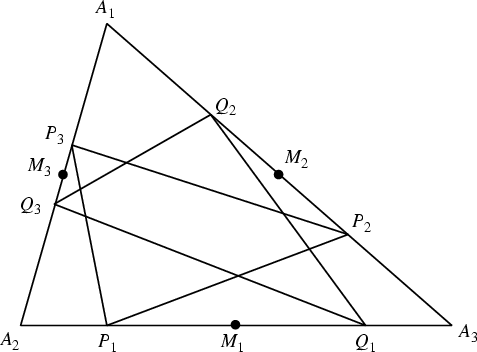Given a Triangle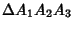with Area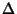, locate the midpoints. Now inscribe two triangles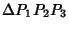and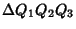with Vertices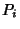and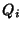placed so that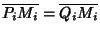. Thenandhave equal areas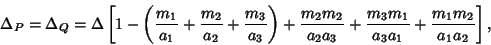where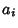are the sides of the original triangle and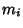are the lengths of the Medians (Johnson 1929).

See also Archimedes' Midpoint Theorem, Brocard Midpoint, Circle-Point Midpoint Theorem, Line Segment, Median (Triangle), Midpoint Ellipse

References

Dunham, W. Journey Through Genius: The Great Theorems of Mathematics. New York: Wiley, pp. 120-121, 1990.

Johnson, R. A. Modern Geometry: An Elementary Treatise on the Geometry of the Triangle and the Circle. Boston, MA: Houghton Mifflin, p. 80, 1929.

Pedoe, D. Circles: A Mathematical View, rev. ed. Washington, DC: Math. Assoc. Amer., 1995.